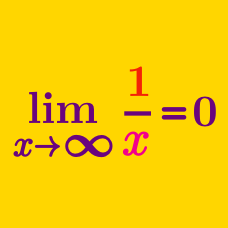Calculus

# Limits of Functions: Level 4 Challenges

$\large \lim_{x \to a} \bigg( 2 - \frac{a}{x} \bigg)^{\tan \frac{\pi x}{2a}} = e^{n}$

Find approximate value of $n$.

$\large \displaystyle \lim_{n \to \infty} \left(\dfrac{a+\sqrt[n]{b}-1}{a}\right)^{n}$

Calculate the limit above in terms of $a$ and $b$, where $a$ and $b$ are constants with $b\geq0$.

Let $f:(1,\infty) \rightarrow (0,\infty)$ be a continuous decreasing function with $\large \lim_{x\to\infty} \dfrac{f(4x)}{f(8x)} = \, 1$

Then

$\large \lim_{x\to\infty} \dfrac{f(6x)}{f(8x)} = \, ?$

Let $\{x_n\}$ be a sequence such that $x_1=1,\ x_nx_{n+1}=2n$ for $n\ge 1$.

Find $\displaystyle \lim_{n\to\infty} \dfrac{|x_{n+1}-x_n|}{\sqrt{n}}.$

Compute $\large \lim_{ n\to{\infty}}{\dfrac{ 2016(1^{2015}+2^{2015}+ \cdots +n^{2015}) - n^{2016}}{2016(1^{2014}+2^{2014}+\cdots+n^{2014})}}.$

×